2019-06-22 19:59:45 qq_43634001 阅读数 521
• ###### 人工智能-机器学习实战视频课程

本课程使用Python3讲解，同时适应于Windows、Mac OS X和Linux。本课程是《机器学习系列课程》中的重要部分。这套视频课程包括但不限于Python基础、常用机器学习框架（如scikit-learn、tensorflow、pytorch、caffe、keras等），机器学习核心算法、大量的实战案例、机器学习的数学基础，机器学习在自然语言处理中的应用、机器学习在推荐系统中的应用。

1690 人正在学习 去看看 李宁

# 机器学习（二）：二分K-means算法

### 二分k-means算法

• 聚合。这是一种自底向上的方法，每一个观察者初始化本身为一类，然后两两结合
• 分裂。这是一种自顶向下的方法，所有观察者初始化为一类，然后递归地分裂它们

### 二分k-means算法的优点

• 二分k-means算法可以加速k-means算法的执行速度，因为它的相似度计算少了
• 能够克服k-means收敛于局部最小的缺点

### 二分k-means算法的步骤

1. 把所有数据初始化为一个簇，将这个簇分为两个簇。
2. 选择满足条件的可以分解的簇。选择条件综合考虑簇的元素个数以及聚类代价（也就是误差平方和SSE），误差平方和的公式如下所示，其中w(i) 表示权重值，y∗该簇所有点的平均值。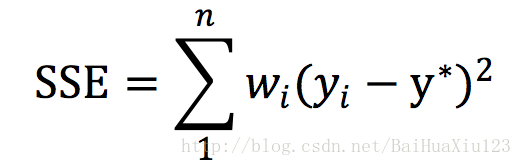3. 使用k-means算法将可分裂的簇分为两簇
4. 一直重复（2）（3）步，直到满足迭代结束条件。

# 二分K-means算法的实践

### 扩展任务

1. 现二分 K-means 代码并进行测试（会在下一节中提到）

### 数据集

Iris.data 数据集主要有如下：

sl 花萼长度
sw 花萼宽度
pl 花瓣长度
pw 花瓣宽度
variety 花的品种

### 实现二分k-means算法

（1) 算法思路：

（2) 算法原理基础：

（3) 算法步骤：

1. 把整个数据集看成一个簇，计算质心
2. 将这个簇分成两个簇
3. 选择满足条件的可以分解的簇，选择条件为簇元素的个数和 SSE 大小
4. 使用 k-mean 算法将可分裂的簇分成两个簇
5. 重复（2）（3）步，直到满足 k 值

（4) 算法相关函数的实现：

• distEclud(): 欧式距离公式
• randCent(): 生成随机 k 个质心
• Kmeans(): k-means 函数
• chooseK(): 画出肘部图
• biKmeans():二分 k-means 的主函数，主要算法
• writeTxt(): 写入文件

（5）代码实现：

# -*- coding:utf-8 -*-
import warnings
import numpy as np
import pandas as pd
import matplotlib.pyplot as plt

warnings.filterwarnings('ignore')   #忽略警告
plt.rcParams['font.sans-serif'] = ['SimHei']  # 用来正常显示中文标签
plt.rcParams['axes.unicode_minus'] = False  # 用来正常显示负号

#伪代码如下
# 将所有点看成一个簇
# 当簇数目小于k时
# 对于每一个簇
#     计算总误差
#     在给定的簇上面进行k-均值聚类（k=2）
#     计算将该簇一分为二之后的总误差
# 选择使得总误差最小的簇进行划分

"""
函数说明：从文件中下载数据，并将分离除连续型变量和标签变量
:parameter:
data - Iris数据集
attributes - 鸢尾花的属性
type - 鸢尾花的类别
sl-花萼长度 , sw-花萼宽度, pl-花瓣长度, pw-花瓣宽度
:return:
"""
iris_data = pd.DataFrame(data=np.array(iris_data), columns=['sl', 'sw', 'pl', 'pw', 'type'], index=range(149))   #给数据集添加列名，方便后面的操作
attributes = iris_data[['sl', 'sw', 'pl', 'pw']]   #分离出花的属性
iris_data['type'] = iris_data['type'].apply(lambda x: x.split('-'))  # 最后类别一列，感觉前面的'Iris-'有点多余即把class这一列的数据按'-'进行切分取切分后的第二个数据
labels = iris_data['type']     #分理出花的类别
attriLabels = []      #建立一个标签列表
for label in labels:        #为了更方便操作，将三中不同的类型分别设为1，2，3
if label == 'setosa':    #如果类别为setosa的话，设为1
attriLabels.append(1)
elif label == 'versicolor':  #如果是versicolor的时候设为2
attriLabels.append(2)
elif label == 'virginica':  #如果是virginica的时候设为3
attriLabels.append(3)
attributes = attributes.values
return attributes, attriLabels

def randCent(dataSet, k):
"""
函数说明：随机初始化k个质心（质心满足数据边界之内）
:param dataSet:数据集
:param k:  质点个数
:return:centroids 返回初始化得到的k个质心向量
"""
m, n = dataSet.shape  # 得到行列数目
centroids = np.zeros((k, n))  # 定义一个k行n列的全为0的质点集合
for j in range(n):
#得到该列数据的最小值
minJ = min(dataSet[:, j])
#得到该列数据的范围
rangeJ = float(max(dataSet[:, j]) - minJ)
#k个质心向量的第j维数据值随机为位于范围内的某一个值
centroids[:, j] = np.mat(minJ + rangeJ * np.random.rand(k))
return centroids

def distEclud(vecA,vecB):
"""
函数说明：欧式距离公式
:param vecA:样本点
:param vecB：质心
:return:距离
"""
return np.sqrt(np.sum((vecA - vecB) ** 2))

def KMeans(dataSet, k, distMeas=distEclud, createCent=randCent):
"""
函数说明：k均值算法
:param dataSet: 特征集
:param k: k个值
:return:centroids, clusterAssment
"""
m = np.shape(dataSet)     #得到行的数目
clusterAssment = np.mat(np.zeros((m, 2))) #初始化一个（m，2）矩阵
clusterChange = True      #定义聚类是否发生变化
centroids = createCent(dataSet, k)        #创建初始化的k个质心向量
while clusterChange:          #只要聚类结果一直发生变化，就一直执行聚类算法，直到所有数据点聚类结果不发生变化
clusterChange = False  #聚类结果定义为False
for i in range(m):     #遍历数据集中的每一个样本
minDist = np.inf       #初始化最小距离为100000
minIndex = -1    #最小距离索引定为-1
for j in range(k): #循环k个类的质心
distance = distMeas(centroids[j, :], dataSet[i, :]) #计算数据点到质心的欧式距离
if distance < minDist:  #如果当前距离少于最小距离
minDist = distance#当前距离定为最小距离，最小距离的索引定为j
minIndex = j
if clusterAssment[i, 0] != minIndex:  #当前聚类结果中第i个样本的结果发生变化
clusterChange = True    #把clusterChange定义为Ture，代表发生了变化
clusterAssment[i, :] = minIndex, minDist**2     #更新当前新变化的聚类结果和错误平方
for j in range(k):                #遍历每一个质心
#因此首先先比较clusterAssment[:,0].A==cent的真假，如果为真则记录了他所在的行，因此在用切片进行取值。
# print(clusterAssment[:, 0].A == j)
if (clusterAssment[:, 0].A == j).all() == False:   #再chooseK防止报错
continue
pointsInCluster = dataSet[np.nonzero(clusterAssment[:, 0].A == j)]
# 计算这些数据的均值（axis=0：求列的均值），作为该类质心向量
centroids[j, :] = np.mean(pointsInCluster, axis=0)
# #返回k个聚类，聚类结果和误差
return centroids, clusterAssment

def biKmeans(dataSet, k, distMeans = distEclud):
"""
函数说明：二分k-均值聚类算法
:param dataSet: 待聚类的数据集
:param k: 聚类的个数
:param distMeans: 用户指定的距离计算方法，这里为欧式距离公式
:return: np.mat(centList)-质心向量
clusterAssment - 聚类结果
"""
m = np.shape(dataSet)    #获得数据集的样本数
clusterAssment = np.mat(np.zeros((m, 2)))   #初始化一个元素全为0的（m，2）的矩阵
centroid0 = np.mean(dataSet, axis=0).tolist()   #获取数据每一列的均值，组成一个一维数组
centList = [centroid0]  #当前聚类列表将数据聚为一类
for j in range(m):  #遍历数据中的每个数据集样本
#计算当前聚类为一类时各个数据点距离质心的平方距离
clusterAssment[j, 1] = distMeans(np.mat(centroid0), np.mat(centroid0)) ** 2
while (len(centList) < k):   #循环，直到达到k类
lowestSSE = np.inf    #将当前最小误差设置为正无穷大
for i in range(len(centList)):  #遍历每个聚类
##因此首先先比较clusterAssment[:,0].A==cent的真假，如果为真则记录了他所在的行，因此在用切片进行取值。
ptsInCurrCluster = dataSet[np.nonzero(clusterAssment[:, 0].A==i), :]
# 对该类利用二分k-均值算法进行划分，返回划分后结果，及误差
centroidMat, splitClustAss = KMeans(ptsInCurrCluster, 2, distMeans)
#计算该划分后两个类的误差平方和
sseSplit = np.sum(splitClustAss[:, 1])
#计算数据集中不属于该类的数据的误差平方和
sseNotSplit = np.sum(clusterAssment[np.nonzero(clusterAssment[:, 0].A!=i), 1])
#划分第i类后总误差小于当前最小总误差
if(sseSplit + sseNotSplit) < lowestSSE:
# 第i类作为本次划分类
bestCentToSplit = i
# 第i类划分后得到的两个质心向量
bestNewCents = centroidMat.copy()
# 复制第i类中数据点的聚类结果即误差值
bestClustAss = splitClustAss.copy()
# 将划分第i类后的总误差作为当前最小误差
lowestSSE = sseSplit + sseNotSplit
# 数组过滤筛选出本次2-均值聚类划分后类编号为1数据点，将这些数据点类编号变为1
# 当前类个数+1，作为新的一个聚类
bestClustAss[np.nonzero(bestClustAss[:, 0].A == 1), 0] = len(centList)
# 同理，将划分数据集中类编号为0的数据点的类编号仍置为被划分的类编号，使类编号
# 连续不出现空缺
bestClustAss[np.nonzero(bestClustAss[:, 0].A == 0), 0] = bestCentToSplit
# # 更新质心列表中的变化后的质心向量
centList[bestCentToSplit] = bestNewCents[0, :]
# 添加新的类的质心向量
centList.append(bestNewCents[1, :])
# 更新clusterAssment列表中参与2-均值聚类数据点变化后的分类编号，及数据该类的误差平方
clusterAssment[np.nonzero(clusterAssment[:, 0].A == bestCentToSplit), :] = bestClustAss.copy()
#返回聚类结果
return np.mat(centList), clusterAssment

def chooseK(dataSet, i):
"""
函数说明：肘部图的绘画
:param dataSet: 数据集
:param i:k从1开始迭代到k次
:return:none
"""
list = []  #定义存放距离的列表，即y轴
x= []      #定义存放1，2，3，4，5，即x轴
for j in range(1, i):
#得到聚点， 聚类结果，和误差
cenList, clusterAssment = KMeans(dataSet, j)
#计算每个点的误差平方和，并加入到列表中
list.append(sum(clusterAssment[:, 1]))
#添加x轴
x.append(j)
#将list转变为列表
a = np.array(list)
list = a.reshape(-1)
#以x为画图
fig = plt.figure()
ax.plot(x, list)
plt.show()

def writeTxt(cenList1, clusterAssment, type=1):
"""
函数说明：将质心和分类结果写入文件的操作。
:param cenList1:质心
:param clusterAssment:分类结果
:param type: 1为二分k-means， 2为k-means
:return: none
"""
#将clusterAssment变成列表
clusterAssment = clusterAssment.tolist()
#获得cenList的长度
n = len(cenList1)
#获得clusterAssment的长度
m = len(clusterAssment)
#判断是否为k-means
if type == 1:
file = open('consequeue01.txt', mode='w')   #如果是二分k-means则打开01文件
file.write("二分k-means的聚类后的质心")
else:
file = open('consequeue02.txt', mode='w')   #如果是k-means则打开02文件
file.write("k-means的聚类后的质心")
for j in range(n):
file.write("\n第%d个质心为：" % (j+1))  #输入质心
file.write(str(cenList1[j]))           #输入质心
file.write("\n聚类结果:\n")
for i in range(m):
file.write('第%d个属性被归类为：' % (i+1))     #输入类别
file.write(str(int(clusterAssment[i])))
file.write("       ")
file.write("距离为：")
file.write(str(clusterAssment[i]))    #输入距离
file.write("\n")
file.close()

#二分k-means算法
if __name__ == '__main__':
filename = "iris.data"      #文件路径
centList, clusterAssment = biKmeans(attributes, 3)   #k=3时得到质心和分类结果
writeTxt(centList, clusterAssment, 1)   #写入文件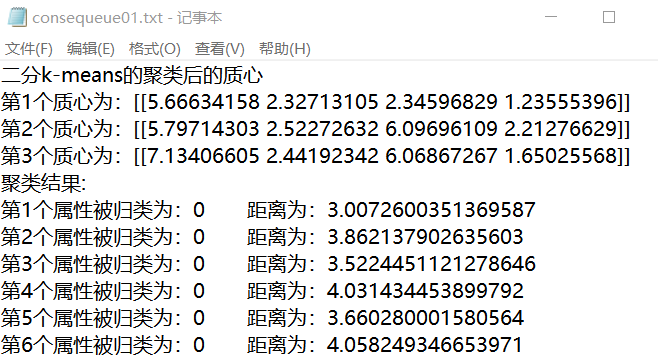k- 机器学习 相关内容

2018-10-29 11:19:49 zhq9695 阅读数 94
• ###### 人工智能-机器学习实战视频课程

本课程使用Python3讲解，同时适应于Windows、Mac OS X和Linux。本课程是《机器学习系列课程》中的重要部分。这套视频课程包括但不限于Python基础、常用机器学习框架（如scikit-learn、tensorflow、pytorch、caffe、keras等），机器学习核心算法、大量的实战案例、机器学习的数学基础，机器学习在自然语言处理中的应用、机器学习在推荐系统中的应用。

1690 人正在学习 去看看 李宁

0. 前言

1. K-means

2. K-means的后处理

3. 二分K-means

4. K 的选择

5. 实战案例

5.1. 原始K-means

5.2. 二分K-means

# 0. 前言

• 监督学习（supervised learning）：训练集合已全部标注所属类别或者目标结果
• 无监督学习（unsupervised learning）：训练集合未进行标注，无法预先知道每个数据的所属类别或者目标结果

• 优点：容易实现
• 缺点：可能收敛到局部最小值，在大规模数据集上收敛较慢
• 适用数据类型：数值型数据

# 1. K-means

K-means， 为用户定义的簇的个数，每一个簇通过其中心（质心）表示。

K-means的算法可表示为：

1. 随机创建  个簇的质心
2. 簇分配：将数据集中每一个点分配到距离最近的簇中
3. 移动聚类中心：重新计算每个簇的中心，更新为该簇所有点的平均值
4. 继续进行簇分配和移动聚类中心，直到没有数据点的分配结果发生改变为止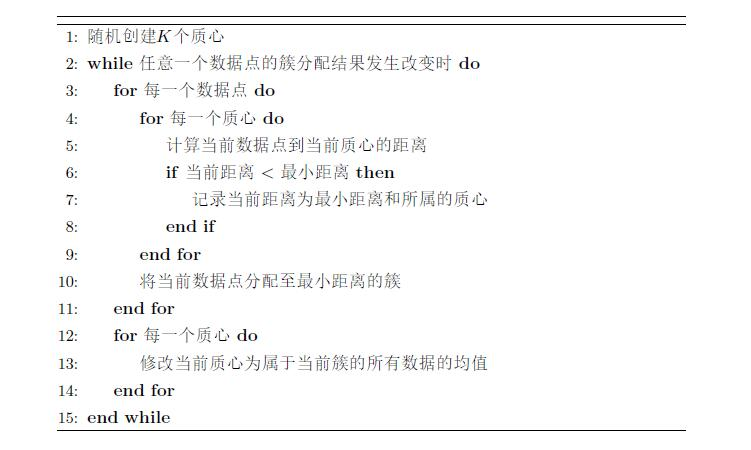# 2. K-means的后处理

K-means聚类算法有时候会陷入局部最优值，如下图所示（图源：吴恩达机器学习）：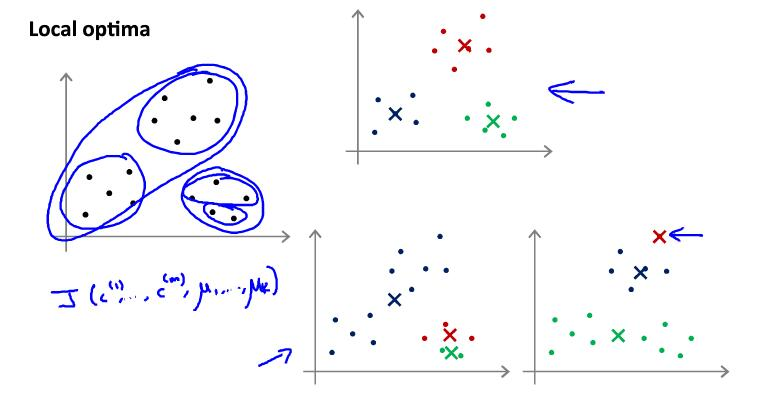1. 可在上述算法外再嵌套一层循环，每次确定簇中心之后计算SSE，多次迭代之后，选择SSE最小的一组结果
2. 将具有最大SSE的簇运用K-means划分为两个簇，然后再合并最近的质心，或者合并两个使SSE增幅最小的质心

# 3. 二分K-means

1. 选择划分后可以最大程度降低SSE的簇
2. 选择当前SSE最大的簇进行划分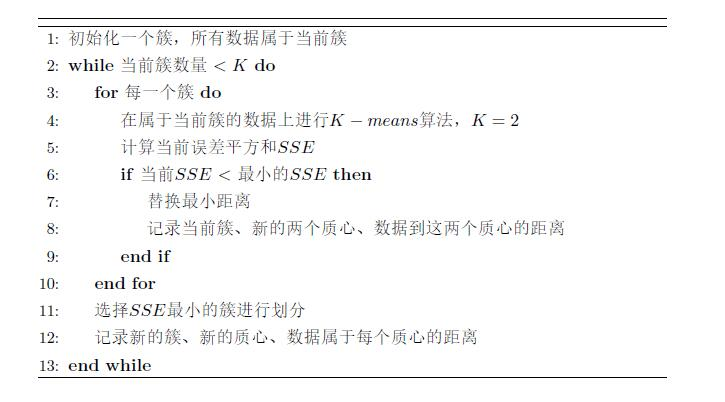# 4. K 的选择

• 人工选择：根据需求或者已知的知识，进行人工选择簇的数量
• 肘部法则：如下图所示（图源：吴恩达机器学习），尝试不同的  ，选择变化率明显变缓的“肘部点”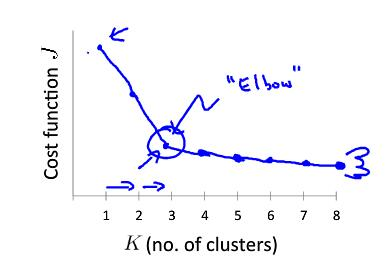# 5. 实战案例

## 5.1. 原始K-means

# coding:utf-8
from numpy import *

"""

"""

# 加载数据集
dataMat = []
fr = open(fileName)
curLine = line.strip().split('\t')
fltLine = list(map(float, curLine))
dataMat.append(fltLine)
return dataMat

# 向量的欧式距离
def distEclud(vecA, vecB):
return sqrt(sum(power(vecA - vecB, 2)))

# 随机生成k个质心
def randCent(dataSet, k):
n = shape(dataSet)
# K个质心的向量
centroids = mat(zeros((k, n)))
for j in range(n):
minJ = min(dataSet[:, j])
rangeJ = float(max(dataSet[:, j]) - minJ)
centroids[:, j] = mat(minJ + rangeJ * random.rand(k, 1))
return centroids

# k-means算法
def kMeans(dataSet, k, distMeas=distEclud, createCent=randCent):
m = shape(dataSet)
# 每个数据对应的簇和距离
clusterAssment = mat(zeros((m, 2)))
# 随机生成k个质心
centroids = createCent(dataSet, k)
clusterChanged = True
while clusterChanged:
clusterChanged = False
# 遍历每一个数据
for i in range(m):
minDist = inf
minIndex = -1
# 遍历每一个质心
for j in range(k):
distJI = distMeas(centroids[j, :], dataSet[i, :])
if distJI < minDist:
minDist = distJI
minIndex = j
# 当前数据的质心改变
if clusterAssment[i, 0] != minIndex:
clusterChanged = True
# 记录当前质心和距离
clusterAssment[i, :] = minIndex, minDist ** 2
# print(centroids)
# 重新计算每个质心的位置
for cent in range(k):
# 获取属于当前簇的数据
ptsInClust = dataSet[nonzero(clusterAssment[:, 0].A == cent)]
# 按列求平均，重新计算质心位置
centroids[cent, :] = mean(ptsInClust, axis=0)
return centroids, clusterAssment

if __name__ == '__main__':
myCentroids, clusterAssing = kMeans(datMat, 4)
print(myCentroids)

## 5.2. 二分K-means

# coding:utf-8
from numpy import *
import matplotlib
import matplotlib.pyplot as plt

"""

"""

# 向量的欧式距离
def distEclud(vecA, vecB):
return sqrt(sum(power(vecA - vecB, 2)))

# 随机生成k个质心
def randCent(dataSet, k):
n = shape(dataSet)
# K个质心的向量
centroids = mat(zeros((k, n)))
for j in range(n):
minJ = min(dataSet[:, j])
rangeJ = float(max(dataSet[:, j]) - minJ)
centroids[:, j] = mat(minJ + rangeJ * random.rand(k, 1))
return centroids

# k-means算法
def kMeans(dataSet, k, distMeas=distEclud, createCent=randCent):
m = shape(dataSet)
# 每个数据对应的簇和距离
clusterAssment = mat(zeros((m, 2)))
# 随机生成k个质心
centroids = createCent(dataSet, k)
clusterChanged = True
while clusterChanged:
clusterChanged = False
# 遍历每一个数据
for i in range(m):
minDist = inf
minIndex = -1
# 遍历每一个质心
for j in range(k):
distJI = distMeas(centroids[j, :], dataSet[i, :])
if distJI < minDist:
minDist = distJI
minIndex = j
# 当前数据的质心改变
if clusterAssment[i, 0] != minIndex:
clusterChanged = True
# 记录当前质心和距离
clusterAssment[i, :] = minIndex, minDist ** 2
# print(centroids)
# 重新计算每个质心的位置
for cent in range(k):
# 获取属于当前簇的数据
ptsInClust = dataSet[nonzero(clusterAssment[:, 0].A == cent)]
# 按列求平均，重新计算质心位置
centroids[cent, :] = mean(ptsInClust, axis=0)
return centroids, clusterAssment

# 二分k-means
def biKmeans(dataSet, k, distMeas=distEclud):
m = shape(dataSet)
# 所有数据属于的簇和与簇质心的距离
clusterAssment = mat(zeros((m, 2)))
# 初始化第一个簇
centroid0 = mean(dataSet, axis=0).tolist()
# 簇列表
centList = [centroid0]
# 计算每一个数据与当前唯一簇的距离
for j in range(m):
clusterAssment[j, 1] = distMeas(mat(centroid0), dataSet[j, :]) ** 2
# 循环执行二分，直到簇的数量达到k
while (len(centList) < k):
lowestSSE = inf
# 遍历每一个簇
for i in range(len(centList)):
# 获取属于当前簇的数据
ptsInCurrCluster = dataSet[nonzero(clusterAssment[:, 0].A == i), :]
# 进行2-means
centroidMat, splitClustAss = kMeans(ptsInCurrCluster, 2, distMeas)
# 计算误差平方和
sseSplit = sum(splitClustAss[:, 1])
sseNotSplit = sum(clusterAssment[nonzero(clusterAssment[:, 0].A != i), 1])
print("sseSplit, and notSplit: ", sseSplit, sseNotSplit)
# 如果小于最小误差，则替换
if (sseSplit + sseNotSplit) < lowestSSE:
# 最佳划分簇
bestCentToSplit = i
# 新的两个簇向量
bestNewCents = centroidMat
# 原先属于最佳划分簇的数据，当前属于的新簇和距离
bestClustAss = splitClustAss.copy()
# 最低的误差平方和
lowestSSE = sseSplit + sseNotSplit
# 将划分后，数据属于新簇的簇索引
# 由0, 1改为bestCentToSplit和len(centList)
bestClustAss[nonzero(bestClustAss[:, 0].A == 1), 0] = len(centList)
bestClustAss[nonzero(bestClustAss[:, 0].A == 0), 0] = bestCentToSplit
print('the bestCentToSplit is: ', bestCentToSplit)
print('the len of bestClustAss is: ', len(bestClustAss))
# 修改被划分的簇的质心，添加新的簇
centList[bestCentToSplit] = bestNewCents[0, :].tolist()
centList.append(bestNewCents[1, :].tolist())
# 取属于被划分簇的那些数据，修改这些数据的新的簇和到簇质心的距离
clusterAssment[nonzero(clusterAssment[:, 0].A == bestCentToSplit), :] = \
bestClustAss  # reassign new clusters, and SSE
return mat(centList), clusterAssment

# 球面余弦定理
def distSLC(vecA, vecB):
a = sin(vecA[0, 1] * pi / 180) * sin(vecB[0, 1] * pi / 180)
b = cos(vecA[0, 1] * pi / 180) * cos(vecB[0, 1] * pi / 180) * \
cos(pi * (vecB[0, 0] - vecA[0, 0]) / 180)
return arccos(a + b) * 6371.0

# 聚类，画图
def clusterClubs(numClust=5):
datList = []
# 加载数据集
lineArr = line.split('\t')
datList.append([float(lineArr), float(lineArr)])
datMat = mat(datList)
# 二分k-means
myCentroids, clustAssing = biKmeans(datMat, numClust, distMeas=distSLC)
# 创建画板
fig = plt.figure()
rect = [0.1, 0.1, 0.8, 0.8]
scatterMarkers = ['s', 'o', '^', '8', 'p', \
'd', 'v', 'h', '>', '<']
axprops = dict(xticks=[], yticks=[])
# 创建底图
# 每一个axes都是一个独立的图层
ax0.imshow(imgP)
# 创建数据图层
# 画每一个簇的数据
for i in range(numClust):
ptsInCurrCluster = datMat[nonzero(clustAssing[:, 0].A == i), :]
markerStyle = scatterMarkers[i % len(scatterMarkers)]
ax1.scatter(ptsInCurrCluster[:, 0].flatten().A, ptsInCurrCluster[:, 1].flatten().A,
marker=markerStyle, s=90)
# 画簇的质心
ax1.scatter(myCentroids[:, 0].flatten().A, myCentroids[:, 1].flatten().A, marker='+', s=300)
plt.show()

if __name__ == '__main__':
clusterClubs(5)


k- 机器学习 相关内容

2018-05-12 17:50:41 XiaoYi_Eric 阅读数 3673
• ###### 人工智能-机器学习实战视频课程

本课程使用Python3讲解，同时适应于Windows、Mac OS X和Linux。本课程是《机器学习系列课程》中的重要部分。这套视频课程包括但不限于Python基础、常用机器学习框架（如scikit-learn、tensorflow、pytorch、caffe、keras等），机器学习核心算法、大量的实战案例、机器学习的数学基础，机器学习在自然语言处理中的应用、机器学习在推荐系统中的应用。

1690 人正在学习 去看看 李宁

### 1.K-Means简介

K均值(K-Means)算法是无监督的聚类方法，实现起来比较简单，聚类效果也比较好，因此应用很广泛。K-Means算法针对不同应用场景，有不同方面的改进。我们从最传统的K-Means算法讲起，然后在此基础上介绍初始化质心优化K-Means++算法，距离计算优化Elkan K-Means算法和大样本情况下Mini Batch K-Means算法。

K-Means算法的思想很简单，对于给定的样本集，按照样本之间的距离大小，将样本集划分为K个簇。让簇内的点尽可能紧密的连在一起，而让簇间的距离尽量的大，下面我们引入K-Means目标函数。

$E=\sum _{i=1}^{k} \sum _{x \in C_i}||x-\mu _i||^2$

$\mu _i=\frac{1}{|C_i|}\sum _{x \in C_i}x$

• 如图(a)所示：表示初始化数据集。
• 如图(b)所示：假设K=2，随机选择两个点作为类别质心，分别为图中的红色质心和蓝色质心。
• 如图(d)所示：对标记为红色的点和蓝色的点分别求新的质心。
• 如图(f)所示：直到质心不再改变后完成迭代，最终得到两个簇C1和C2。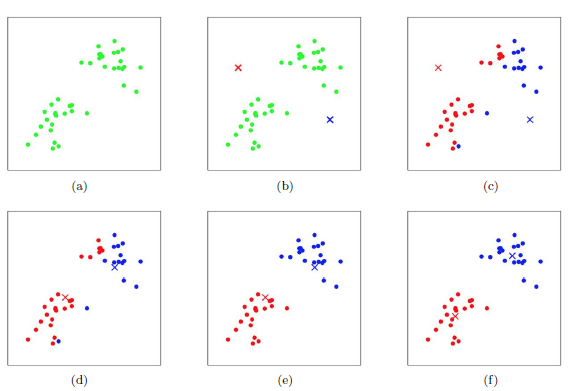### 2.K-Means算法流程

• 从数据集D中随机选择K个样本作为初始的质心向量$\mu={ \mu_1,\mu_2,\mu_3,…,\mu_k }$。

• 迭代$n=1,2,…,N$

• 划分初始化簇$C_t=\varnothing ;\ t=1,2,…,k$
• 对于$i=1,2,…,m$，计算样本$x_i$和各个质心向量$\mu_j(\ j=1,2,…,k)$的距离$d_{ij}$。将$x_i$标记为最小的$d_{ij}$所对应的类别$\lambda_i$，此时更新$C_{\lambda i}=C_{\lambda i} \cup{x_i}$

$d_{ij}=||x_i-\mu_j||^2$

• 对于$j=1,2,…,k$，对$C_j$中所有样本点重新计算新的质心。

$\mu_j=\frac{1}{|C_j|}\sum_{x\in C_j}x$

• 如果K个质心向量都不再发生变化，则结束迭代。
• 输出K个划分簇$C$$C=\{C_1,C_2,C_3,…,C_k \}$

• **对于K值的选择:**我们可以通过对数据的先验经验选择合适的K值，如果没有先验条件的话，还可以通过交叉验证选择合适的K值。
• **对于K个初始化质心:**由于我们采用启发式迭代方法，K个初始化质心的位置选择对最后的聚类结果和运行时间都有较大的影响，最好选择的K个质心不要离得太近。

### 3.初始化优化K-Means++

• 从输入的数据点集合中随机选择一个点作为第一个聚类中心μ1。
• 对于数据集中的每个点xi，计算与他最近的聚类中心距离。

$D(x)=\arg \min_{r=1}^{k_{selected}}||x_i-\mu_r||^2$

• 选择一个数据点作为新的聚类中心，其中D(x)较大的点被选作新的聚类中心的概率较大。
• 重复上述两步，直到选择出K个聚类中心。然后利用这K个质心来作为初始化质心去运行传统K-Means算法。

### 4.距离计算优化Elkan K-Means算法

• 对于一个样本点$x$和两个质心$\mu_{j1},\mu_{j2}$，如果我们预先计算出这两个质心之间的距离$D(j_1,j_2)$，如果发现$2D(x,j_1)≤D(j_1,j_2)$，那么我们便能得到$D(x,j_1)≤D(x,j_2)$。此时我们不再计算$D(x,j_2)$，也就节省了一步距离计算。
• 对于一个样本点$x$和两个质心$μ_{j1},μ_{j2}$，我们能够得到$D(x,j_2)≥max{0,D(x,j_1)−D(j_1,j_2)}$

Elkan K-Means迭代速度比传统K-Means算法迭代速度有较大提高，但如果我们的样本特征是稀疏的，或者有缺失值的话，此种方法便不再使用。

### 5.大样本优化Mini Batch K-Means算法

Mini Batch K-Means采用无放回随机采样的方法从样本集中选取部分数据，然后用选取的数据进行传统的K-Means算法训练。然后进行迭代并更新质心，直到质心稳定或达到指定的迭代次数。

Mini Batch K-Means可以避免样本量太大带来的计算问题，算法收敛速度也能够加快，当然带来的代价就是我们的聚类精确度降低。为增加算法的准确性，我们可以多训练几次Mini Batch K-Means算法，用不同的随机采样集来得到聚类簇，选择其中最优的聚类簇。

### 6.Sklearn实现K-Means算法

from sklearn.cluster import KMeans
import matplotlib.pyplot as plt

X=iris.data[:,:2]
print(X.shape)
#150,2

#plot data
plt.figure()
plt.scatter(X[:,0],X[:,1],c='blue',
marker='o',label='point')
plt.legend(loc=2)
plt.show()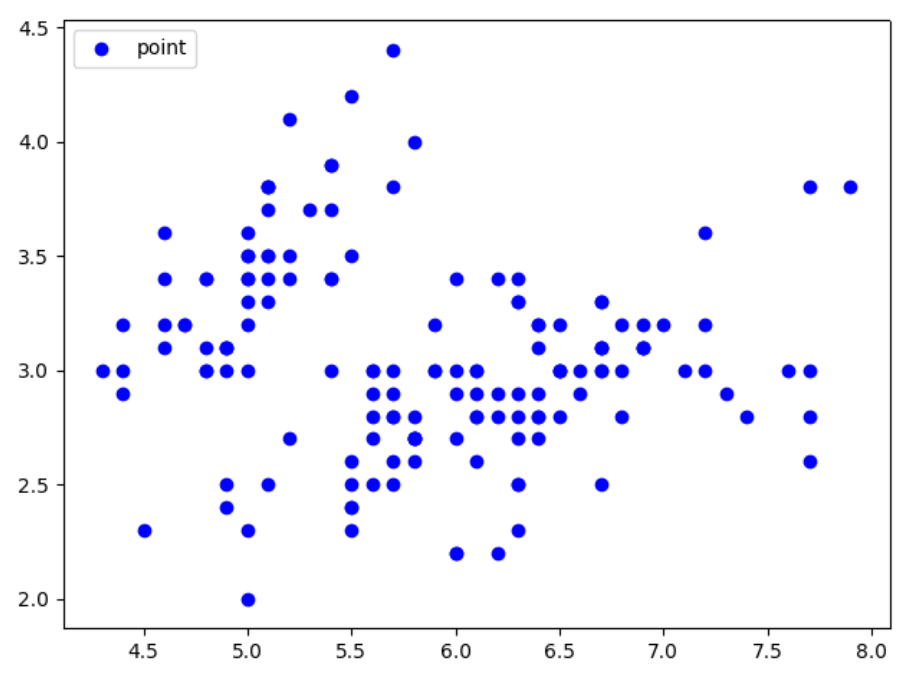# fit data
kmeans=KMeans(n_clusters=3)
kmeans.fit(X)
label_pred=kmeans.labels_

plt.figure()
x0 = X[label_pred == 0]
x1 = X[label_pred == 1]
x2 = X[label_pred == 2]
plt.scatter(x0[:, 0], x0[:, 1], c = "red",
marker='o', label='label0')
plt.scatter(x1[:, 0], x1[:, 1], c = "green",
marker='*', label='label1')
plt.scatter(x2[:, 0], x2[:, 1], c = "blue",
marker='+', label='label2')
plt.legend(loc=2)
plt.show()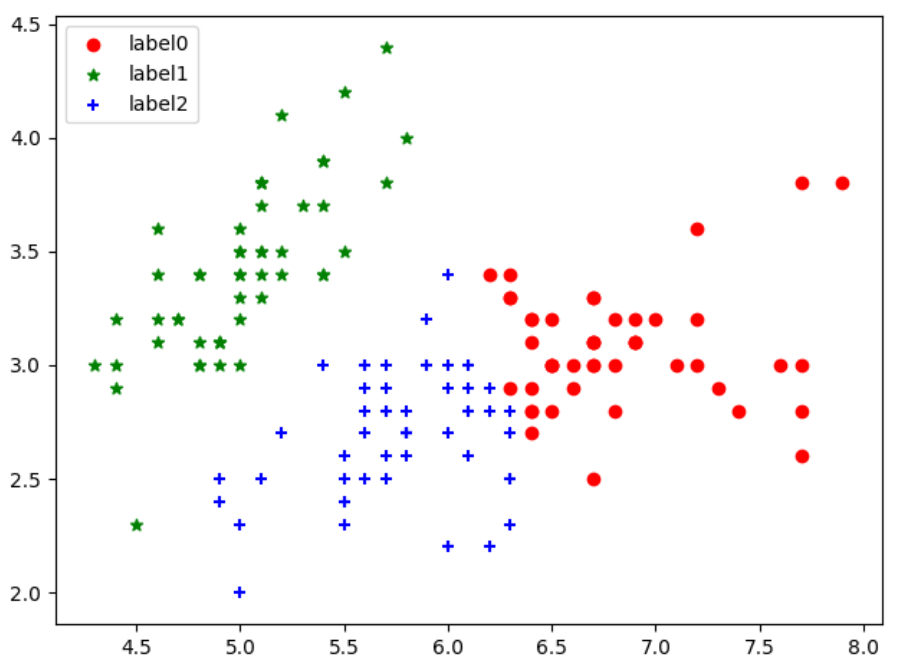### 7.K-Means算法优缺点

#### 7.1优点

• 聚类效果较优。

• 原理简单，实现容易，收敛速度快。

• 需要调整的参数较少，通常只需要调整簇数K。

#### 7.2缺点

• K值选取不好把握。
• 对噪音和异常点比较敏感。
• 采用迭代方法，得到的结果是局部最优。
• 如果各隐含类别的数据不平衡，则聚类效果不佳。

### 8.推广k- 机器学习 相关内容

2019-07-17 09:08:49 qq_37665301 阅读数 34
• ###### 人工智能-机器学习实战视频课程

本课程使用Python3讲解，同时适应于Windows、Mac OS X和Linux。本课程是《机器学习系列课程》中的重要部分。这套视频课程包括但不限于Python基础、常用机器学习框架（如scikit-learn、tensorflow、pytorch、caffe、keras等），机器学习核心算法、大量的实战案例、机器学习的数学基础，机器学习在自然语言处理中的应用、机器学习在推荐系统中的应用。

1690 人正在学习 去看看 李宁

## k-means算法

### 简介

k-means算法是一种聚类算法，所谓聚类，即根据相似性原则，将具有较高相似度的数据对象划分至同一类簇，将具有较高相异度的数据对象划分至不同类簇。聚类与分类最大的区别在于，聚类过程为无监督过程，即待处理数据对象没有任何先验知识，而分类过程为有监督过程，即存在有先验知识的训练数据集。
由于k-means聚类算法需要检测两个对象间的相似性，但对象数据是无标签的，因此很难定义相似性。我们可以按照自己的实验目的定义不同的相似性规则，然后按照规则去计算数据对象之间的相似性。
比如，假设数据对象集是一组二维数据，{(xi,yi)，0<i<N}。假设这些数据中有k (k<N) 个数据是精确的，其余数据分别是这k个数据的近似值，则当我们希望将这些数据分为k组且每组包含一个精确数据和其对应的近似值时 (并不是求出k个精确值，因为无标签，我们不知道那些数据是精确的)，数据对象之间的相似性可定义为对象数据之间的欧式距离*(两点间的距离)*，欧式距离越小，则相似性越高，数据之间越相近，更有可能划分到同一组，欧式距离越大，则相似性越小，数据之间越远，更有可能被划分到不同组中，需要注意的是当这k个数据之间的欧式距离很小时，这种相似性定义方法不能帮助我们得到正确的k个分组。假设数据对象中的x表示时间，当我们想要将对应时间相近的数据对象分到一组时，我们不需要再考虑y，此时相似性可定义为x之间的差距|xi-xj|。

### 种类

1. 水平聚类：分区彼此独立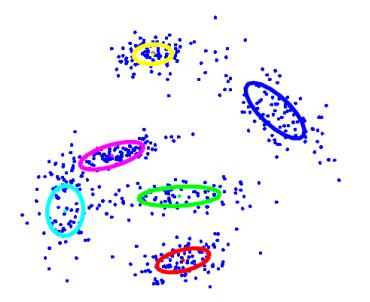2. 层次聚类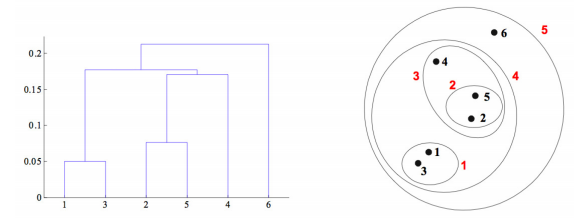### 原理

假设需要将数据分为k个类，以欧式距离作为相似性评判标准。

1. 随机选取k个数据作为初始k个类簇的质心。
2. 将数据集中的每个数据di ( di=(xi,yi) ) 拿出来与每个质心计算欧式距离，如果di与质心kj的欧式距离最小，则将di划分到第j个类簇中。
3. 计算每个类簇中的平均值，将此平均值作为此类簇的新的质心。
4. 如果每个类簇中新质心与之前的质心差值足够小，则将k个类簇作为k个组输出；如果新质心与之前的质心差值不满足要求，则转到第2步进行迭代更新。

### 运行过程示例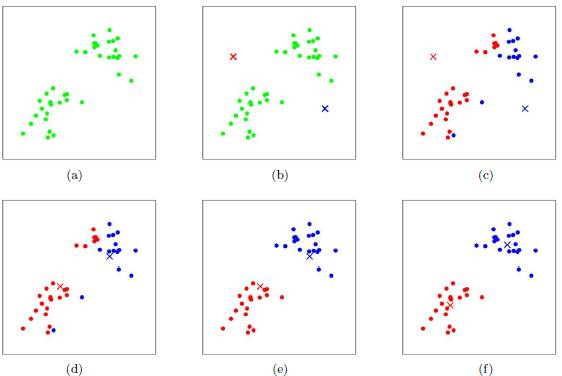### 目标

通过k-means聚类算法，我们得到了k个组，我们希望组内数据之间相似度较高，组间数据之间相似度较低。

### 特点

1. 优点：算法实现简单
2. 缺点：需要用户指定分组的个数； 聚类结果对初始类簇中心的选取较为敏感； 容易陷入局部最优状况。

## k-means算法实验

### 实验内容

1.描述
在这个练习中，你将使用k-means算法通过减少图像包含的颜色数量来压缩图像。首先，下载data6.zip并将其内容加载到Matlab/Octave工作目录中。
照片来源：本练习使用的bird照片属于Frank Wouters，经其许可使用。
2. 图像表示
这个练习的数据包含一个538像素×538像素的TIFF图像，名为bird_large.tiff。它看起来像下面的图片。这幅图像的直接24位颜色表示中，每个像素由三个8位数字(从0到255)表示，它们指定了红、绿和蓝的强度值。我们的这张照片包含了上千种颜色，但我们想把这个数字减少到16种。通过这种减少，只存储图像中16种颜色的RGB值，这样我们可以更高效地表示这张照片。
在这个练习中，您将使用k-means算法将颜色数量减少到k=16。也就是说，你将计算16种颜色作为聚类中心，并将图像中的每个像素替换为最近的聚类中心颜色。
因为计算聚类538×538图像对电脑很耗时，你将在128×128的bird_small.tiff上运行k-means算法。一旦你得到了小图像上的聚类中心，就可以使用这16种聚类中心颜色替换大图像中的像素。
3. K-means in Matlab/Octave
（1）在Matlab/Octave中，用以下命令将小图像加载到程序中：

A = double(imread('bird_small.tiff'));


这将创建一个三维矩阵A，其前两个索引标识像素位置，最后一个索引表示红色、绿色或蓝色。例如，A(50,33,3)给出了像素在位置y=50，x=33处的蓝色强度。（首先给出Y位置，但在我们的示例中这并不重要，因为X和Y尺寸具有相同的尺寸）。
你的任务是从该图像计算16个类簇中心，每个中心是一个长度为3的向量，包含一组RGB值。k-means算法适用于解决这个问题。
（2）当k个聚类中心收敛后，将大图像加载到程序中，并用从小图像中找到的最接近质心的颜色替换其每个像素颜色。
重新计算大图像后，可以按以下方式显示和保存图像:

%Display
imshow(uint8(round(large_image)));
%Save image
imwrite(uint8(round(large_image)),'bird_kmeans.tiff');


### 实验代码

small = double(imread('E:\machine_learning\experiment\exp6\data6\ex8Data\bird_small.tiff'));
%16个中心的颜色
cr = zeros(1,16);
cg = zeros(1,16);
cb = zeros(1,16);
size_small = size(small,1);
%伪随机取16种颜色
for i=1:16
position = 7*i;
cr(1,i) = small(position,position,1);
cg(1,i) = small(position,position,2);
cb(1,i) = small(position,position,3);
end

%为每个像素点初始一个空间，保存其对应个类簇号
s_matrix = zeros(size_small, size_small);
%获取16个聚类
%迭代100次，100次时两个数据中心距离足够近。
for num = 1:100
for j=1:128
for k=1:128
cmin = inf;%像素颜色数据与16个聚类中心最小的距离,初始为无穷大
for i=1:16
r = small(j,k,1);
g = small(j,k,2);
b = small(j,k,3);
dr = r-cr(1,i);
dg = g-cg(1,i);
db = b-cb(1,i);
c = dr*dr+dg*dg+db*db;%距离,没有求根，因为只比较大小，对具体数值无要求
if cmin > c
cmin = c;%更新最小距离
s_matrix(j,k) = i;%更新所属类簇号
end
end
end
end

%更新聚类中心
count = zeros(1,16);
for k = 1:16
for i=1:128
for j=1:128
if(s_matrix(i,j) == k)
count(1,k) = count(1,k)+1;%计算类中有几个点
end
end
end
end
red = zeros(1,16);
green = zeros(1,16);
blue = zeros(1,16);
for k = 1:16
for i=1:128
for j=1:128
if(s_matrix(i,j) == k)%将一个类中的所有值都加起来
red(1,k) = red(1,k) + small(i,j,1);
green(1,k) = green(1,k) + small(i,j,2);
blue(1,k) = blue(1,k) + small(i,j,3);
end
end
end
cr(1,k) = red(1,k)/count(1,k);%求平均数，来作为新的聚类中心
cg(1,k) = green(1,k)/count(1,k);
cb(1,k) = blue(1,k)/count(1,k);
end
end

%压缩small图片
for k = 1:16
for i = 1:size_small
for j =1:size_small
if(s_matrix(i,j) == k)
small(i,j,1) = cr(1,k);
small(i,j,2) = cg(1,k);
small(i,j,3) = cb(1,k);
end
end
end
end
%显示图片
%imshow(uint8(round(small)));
%保存图片
imwrite(uint8(round(small)),'bird_small.jpg');

%压缩large图片
size_large = size(large, 1);
l_matrix = zeros(size_large, size_large);
for i = 1:size_large
for j =1:size_large
dmin = inf;
for k=1:16
dr = large(i,j,1)-cr(1,k);
dg = large(i,j,2)-cg(1,k);
db = large(i,j,3)-cb(1,k);
distance = dr*dr+dg*dg+db*db;
if dmin > distance
dmin = distance;
l_matrix(i,j) = k;
end
end
end
end
for k = 1:16
for i = 1:size_large
for j =1:size_large
if(l_matrix(i,j) == k)
large(i,j,1) = cr(1,k);
large(i,j,2) = cg(1,k);
large(i,j,3) = cb(1,k);
end
end
end
end
%显示图片
imshow(uint8(round(large)));
%保存图片
imwrite(uint8(round(large)),'bird_large.jpg');


### 实验结果

原图像：压缩后的图像### 实验数据和代码

k- 机器学习 相关内容

2018-09-25 20:05:36 u014351944 阅读数 104
• ###### 人工智能-机器学习实战视频课程

本课程使用Python3讲解，同时适应于Windows、Mac OS X和Linux。本课程是《机器学习系列课程》中的重要部分。这套视频课程包括但不限于Python基础、常用机器学习框架（如scikit-learn、tensorflow、pytorch、caffe、keras等），机器学习核心算法、大量的实战案例、机器学习的数学基础，机器学习在自然语言处理中的应用、机器学习在推荐系统中的应用。

1690 人正在学习 去看看 李宁

### Clustering

#### K-Mean Algorithm

下图中已经有没有标签的点，现在需要分为两类
进行k-Mean算法
1、随机选取两个聚类中心，需要分为几类就选取几个聚类中心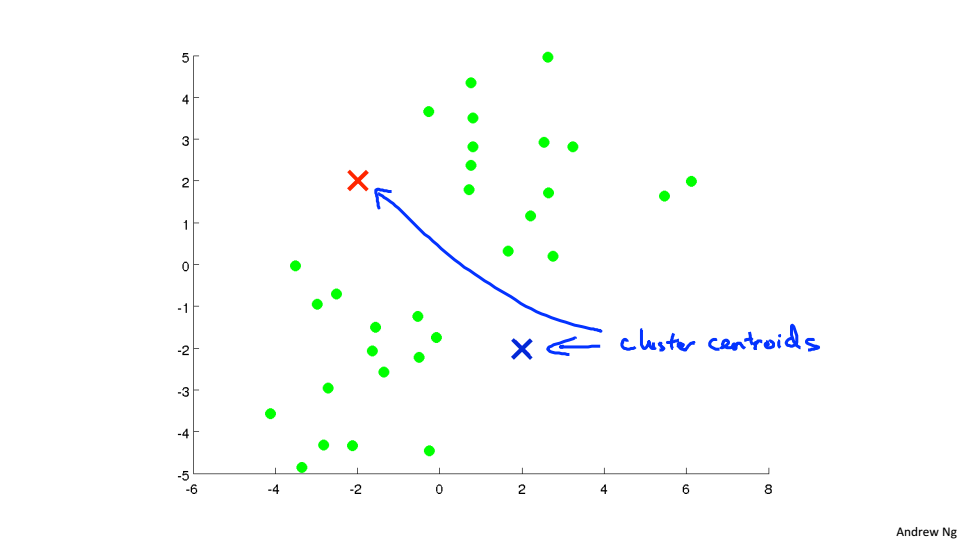2、遍历所有的点，根据点到聚类中心的距离来判断将该点分到哪个聚类中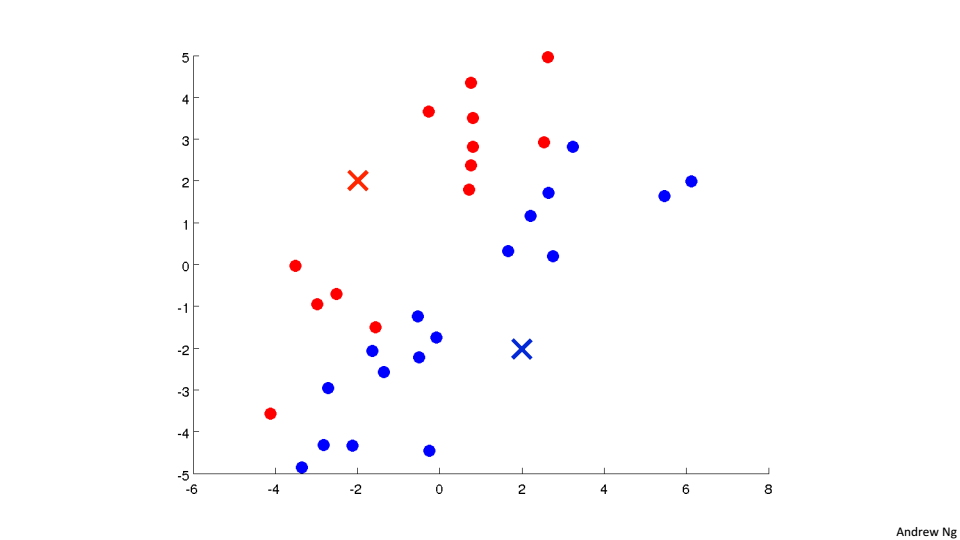3、然后将红色的聚类中心移动到所有红色点的均值位置，蓝色的聚类中心移动到所有蓝色点的均值位置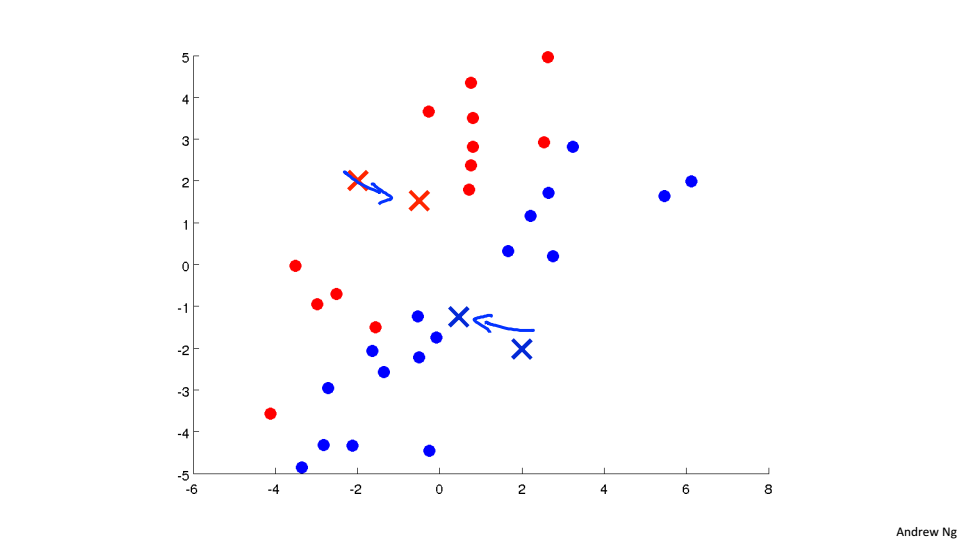重复2、3过程直到收敛
K-Mean中的K就只的是要分为几类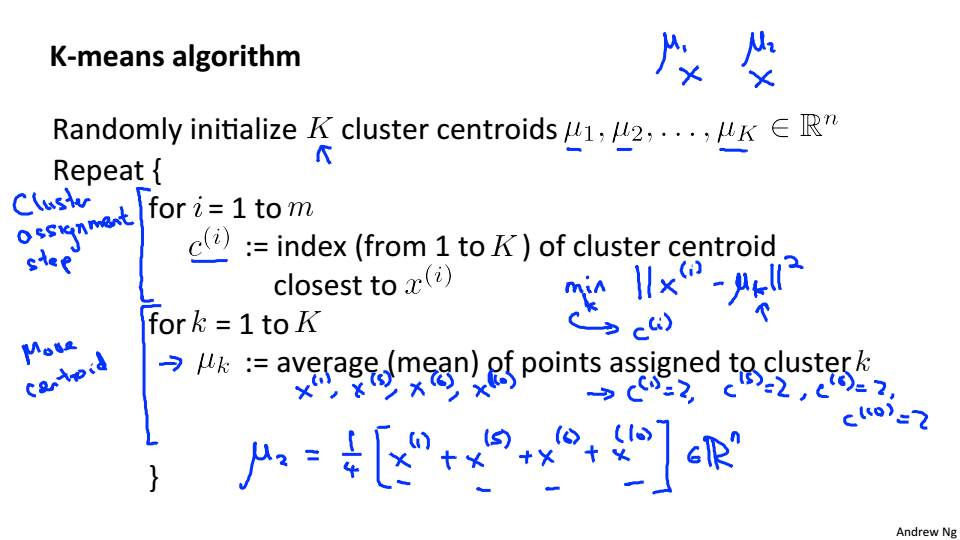#### Opetimization objective

K-Mean中的优化目标
J是点到聚类中心的平均距离，J也被称为失真函数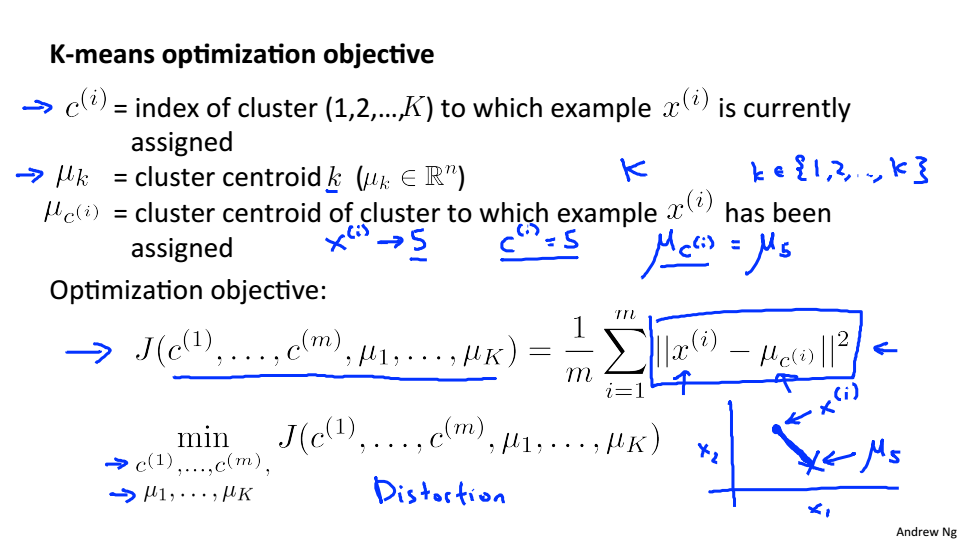#### Random Initialization

随机选取聚类中心
一般是随机选取K个样本，在让聚类中心等于这些样本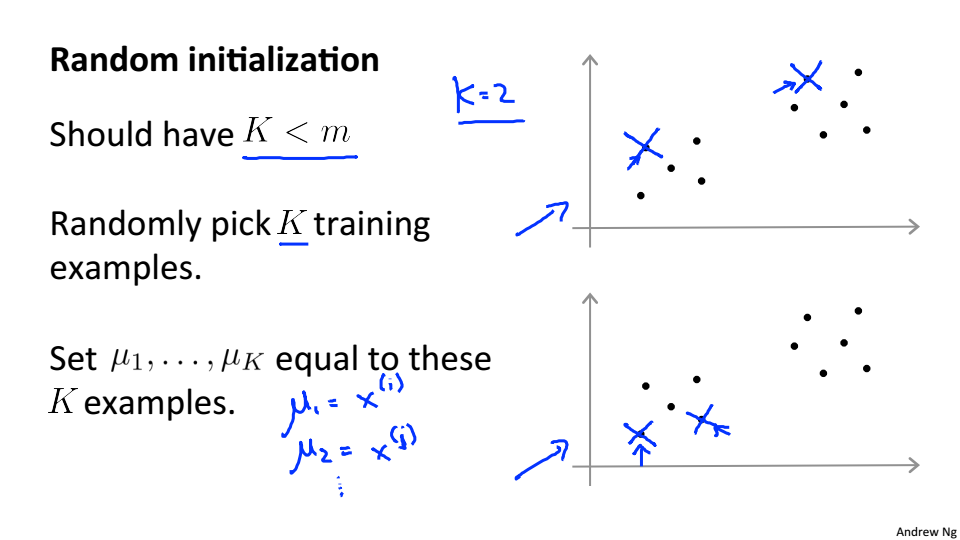局部最优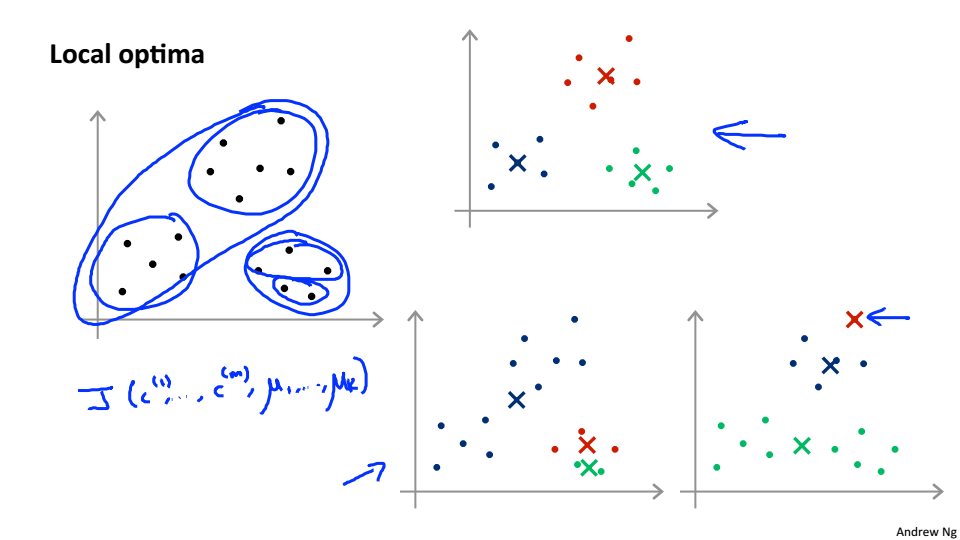加入随机初始化和代价函数后的K-Mean,最后的J的结果一般在2~10之间才是全局最优解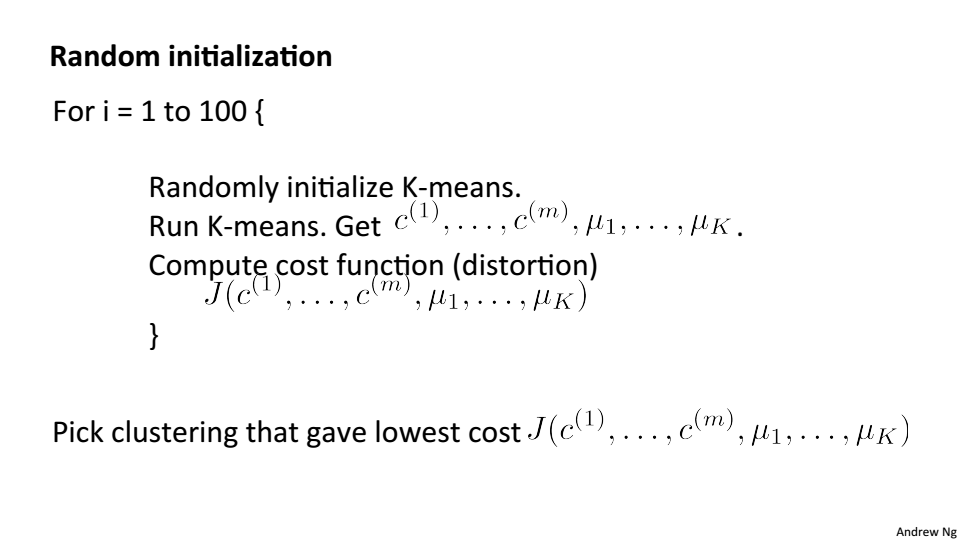#### Choosing the number of Clusters

Elbow Method
绘制代价函数关于K的函数曲线,J会随着K的增大而减小，逐渐趋于平稳，拐点处的K即我们所需要的K
但大部分情况拐点不是很清晰，不能很明显的找出来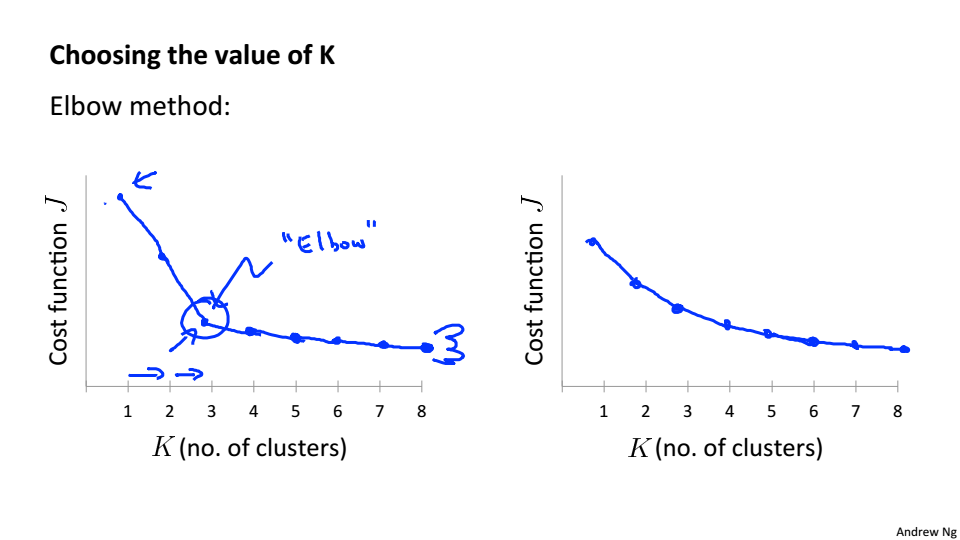通过聚类要达到的目的来确定K的值，以T恤为例，如果T恤的尺码只有S、M、L那么K就应该选择3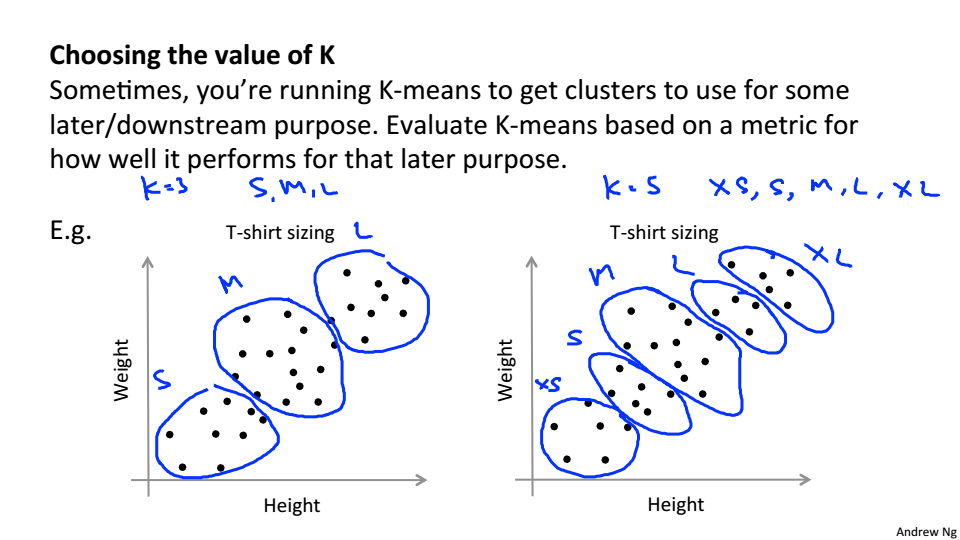### Motivation

#### Data Compression

数据压缩不仅可以使数据量减少，减小内存和硬盘的占用，而且可以提高算法的计算速度
数据压缩就是寻找相关特征之间的关系如下图中的x1和x2都是表示长度，只是单位不一样，就可以根据他们的线性关系，合并为一个一维特征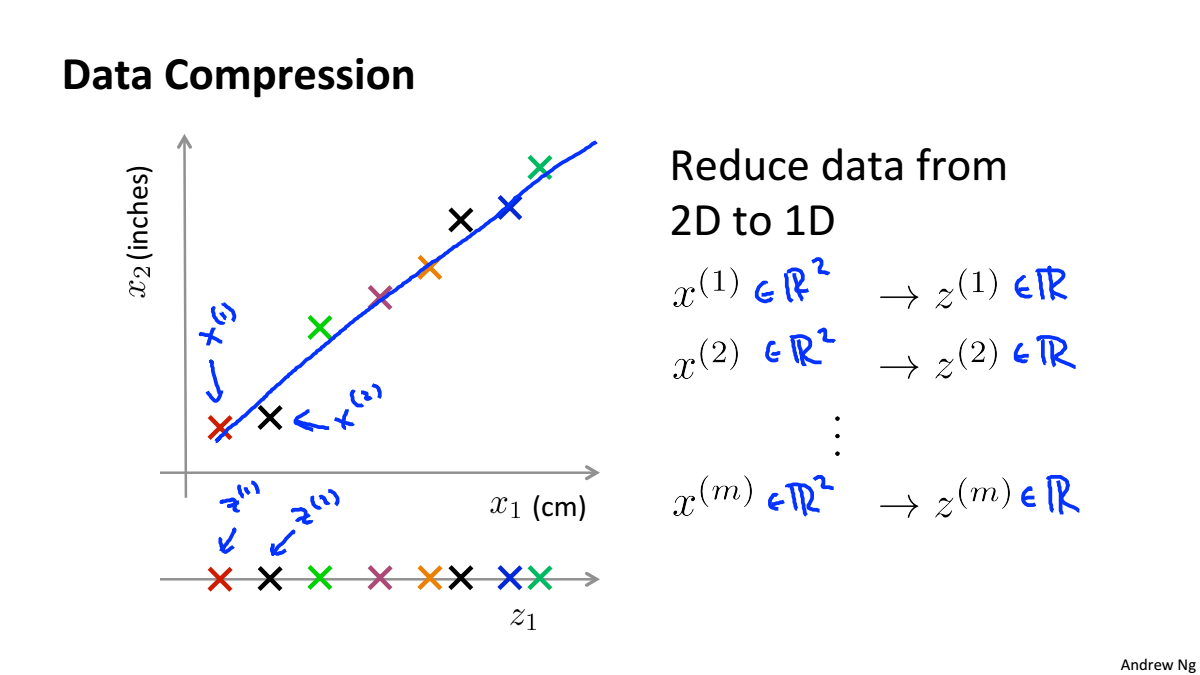三维数据降到二维
将三维数据投影到一个二维平面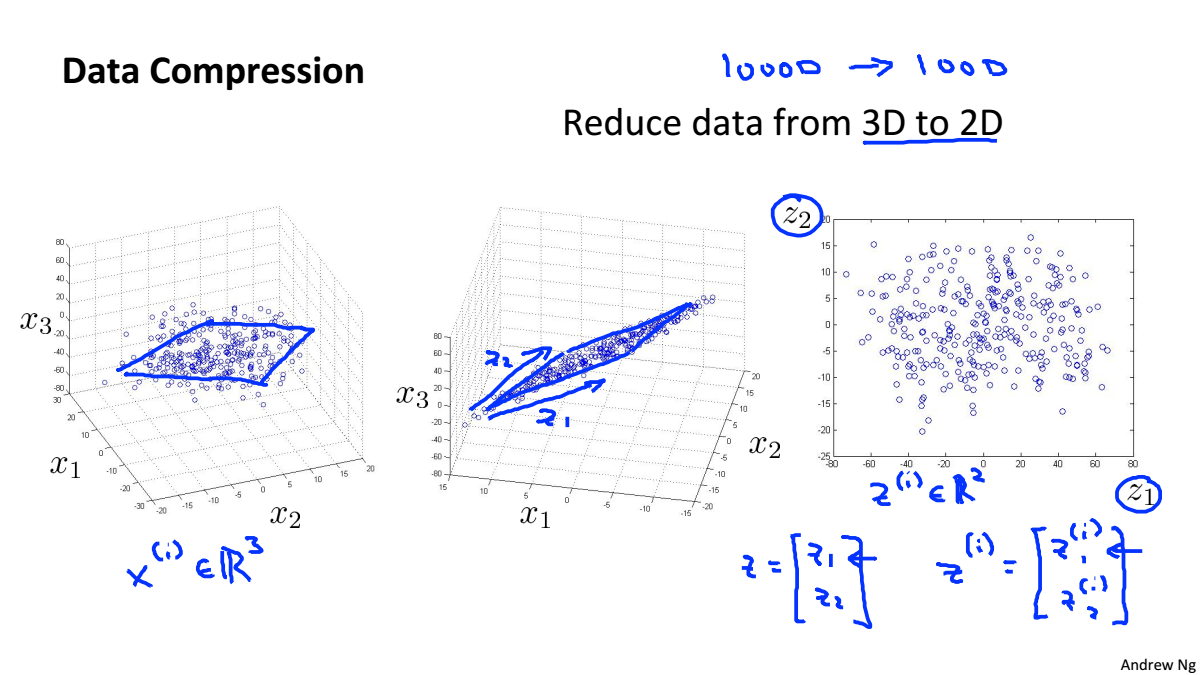#### Data Visualiztion

一个高维数据可以被计算机处理，但人只能感知三维的数据，所以在做高维数据可视化时，要先对数据进行降维，在进行可视化。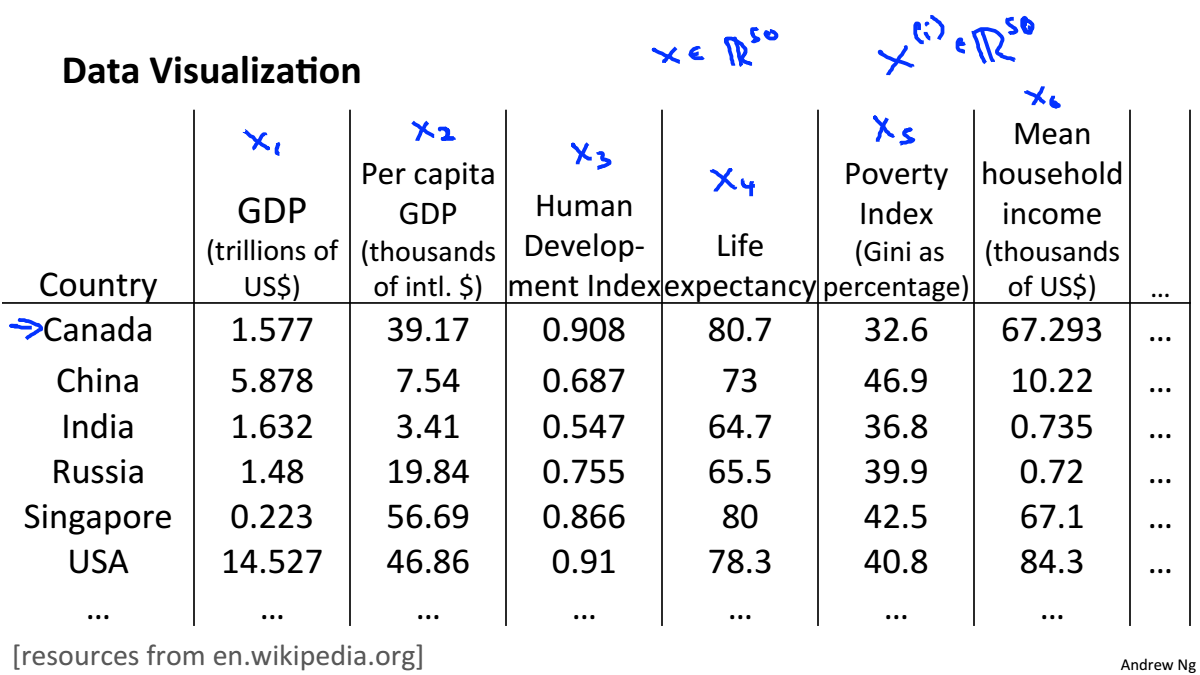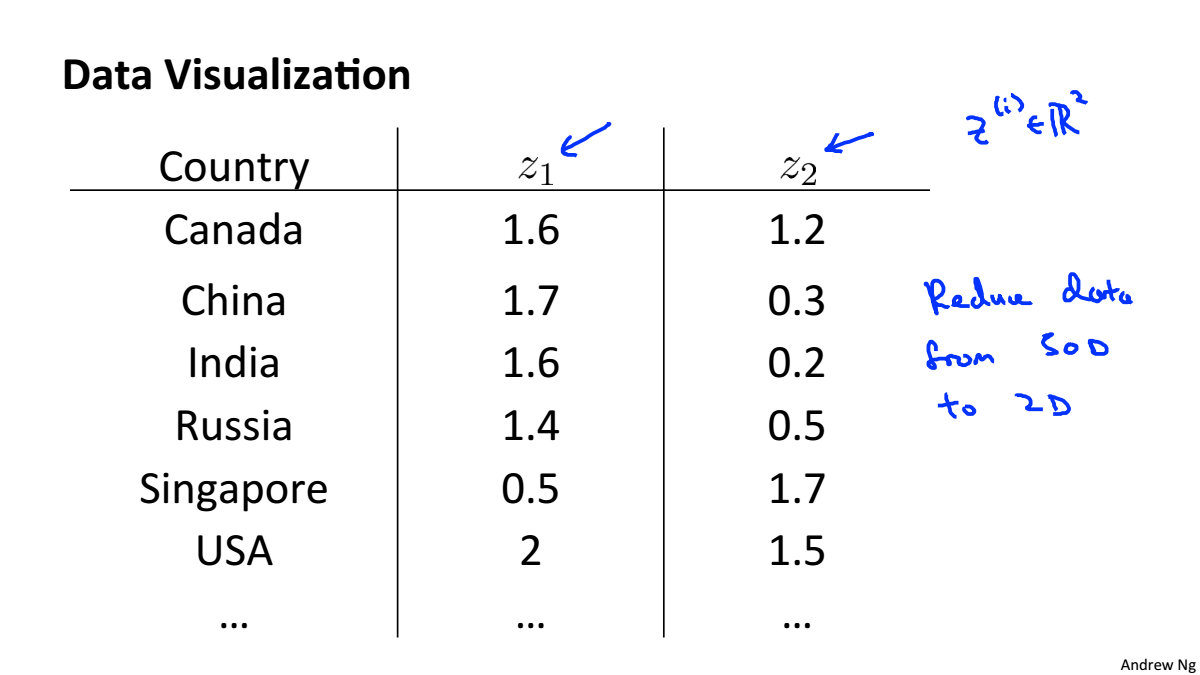### Principal Component Analysis

#### Principal Component Analysis Problem Formulation

PCA将高维数据投影到低维空间,使得数据投影到这个面的误差最小,这个误差也叫作投影误差，在进行降维之前，要对数据进行均值归一化和特征规范化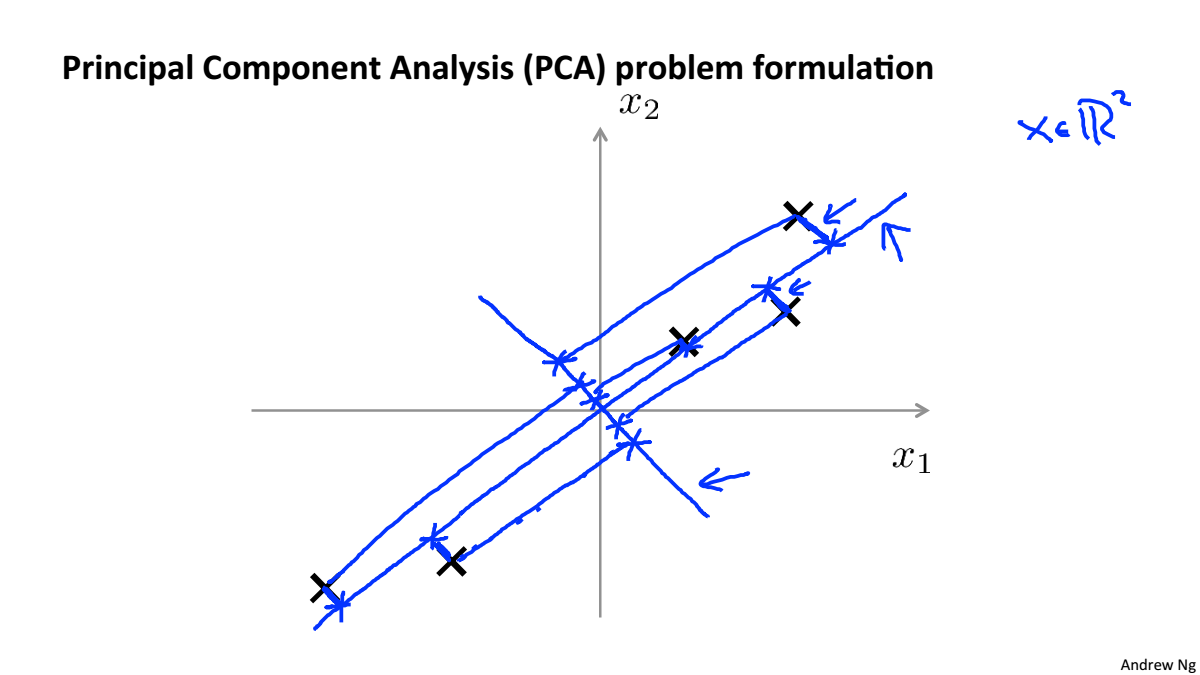对于二维降到一维，是要寻找一个向量，使得投影误差最小
对于n维降到k维，是要寻找k个向量，使得投影误差最小
PCA和线性回归的区别：

1. 线性回归的误差是结果值得误差，PCA是点到投影面的距离
2. PCA是无监督学习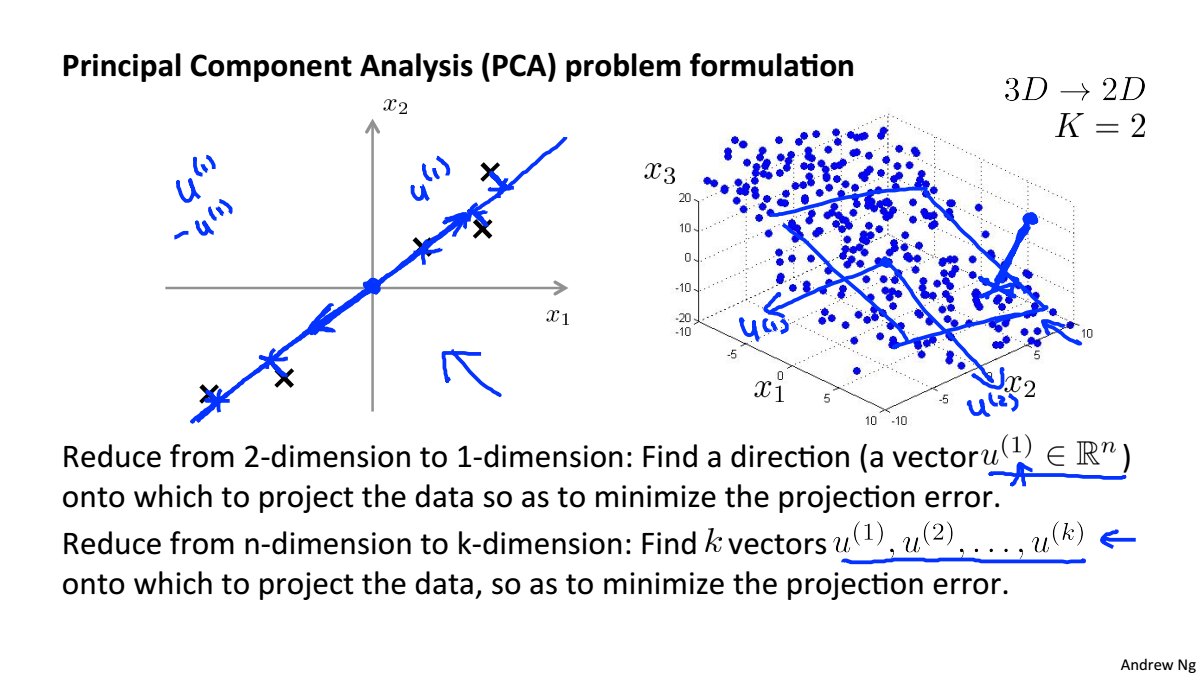#### Principal Component Analysis Algorithm

数据预处理(特征缩放/均值归一化)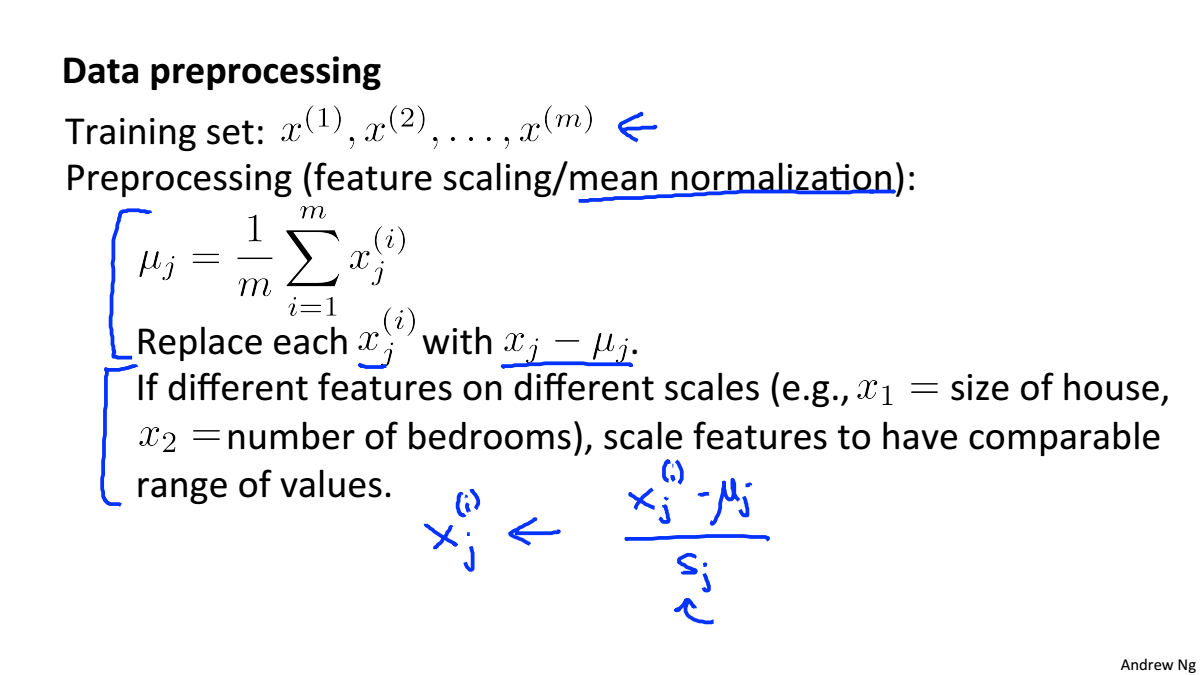PCA算法的步骤：

1. 计算协方差矩阵
2. 使用svd计算$\Sigma$的特征值和特征向量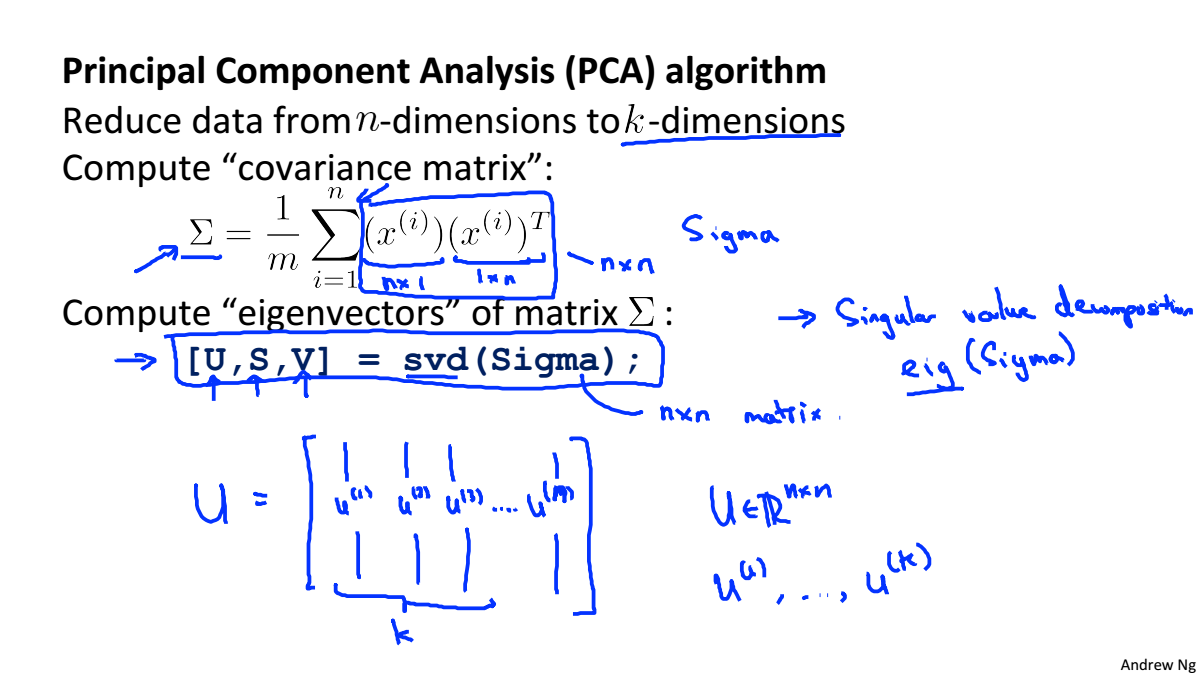3. 将特征向量按对应特征值大小从上到下按行排列成矩阵，取前k行组成矩阵$U_r$
4. $Z=U^T*X$就是降维之后的数据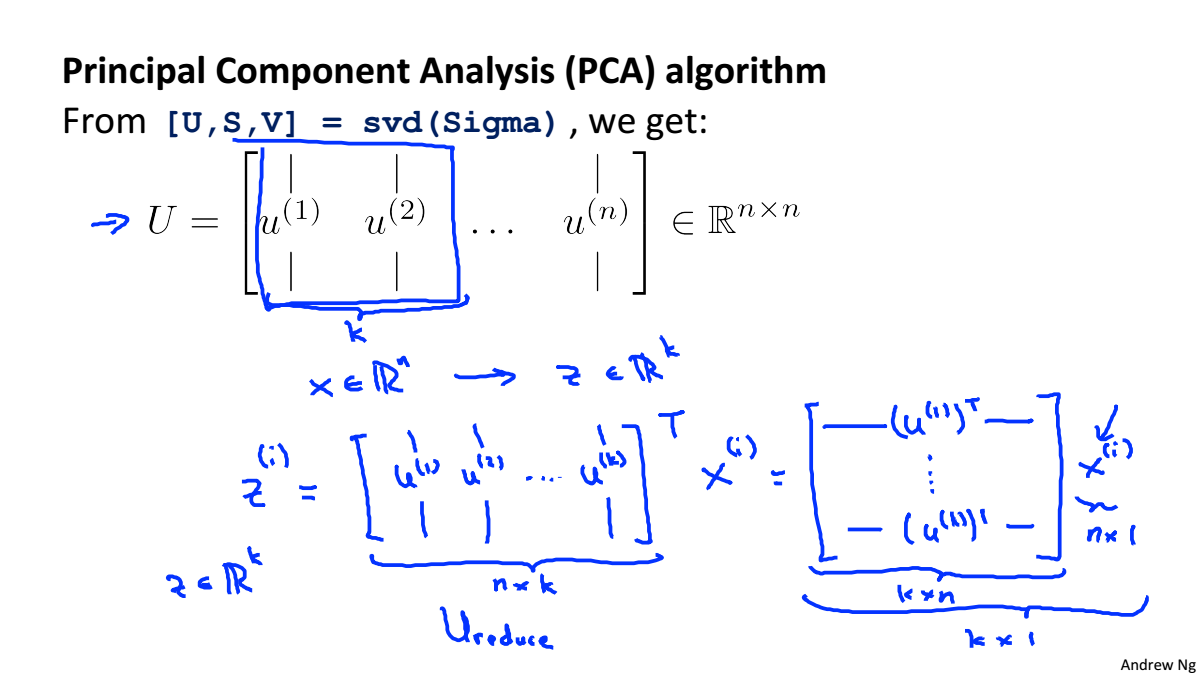### Applying PCA

#### Reconstruction from Compressed Representation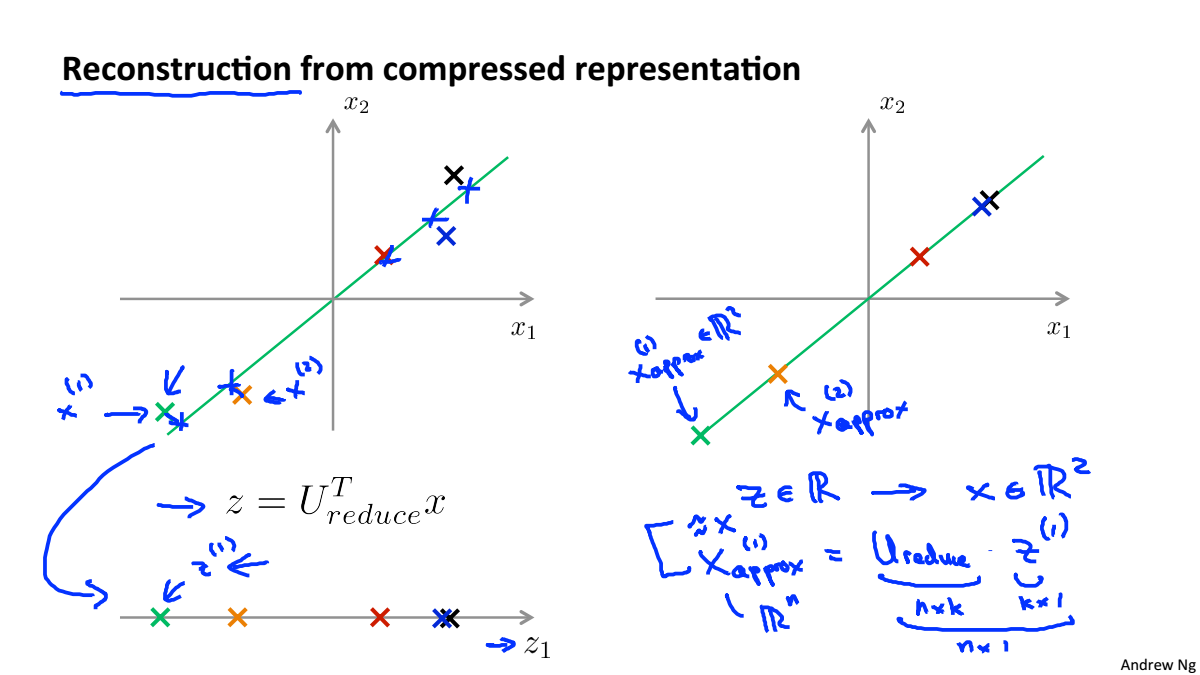#### Choosing the Number of Principal Components

计算平均投影误差和总变差的比，选择该值最小时候的K，该值表示的是与原数据的差异性，当为0.01时代表PCA保留了99%的差异性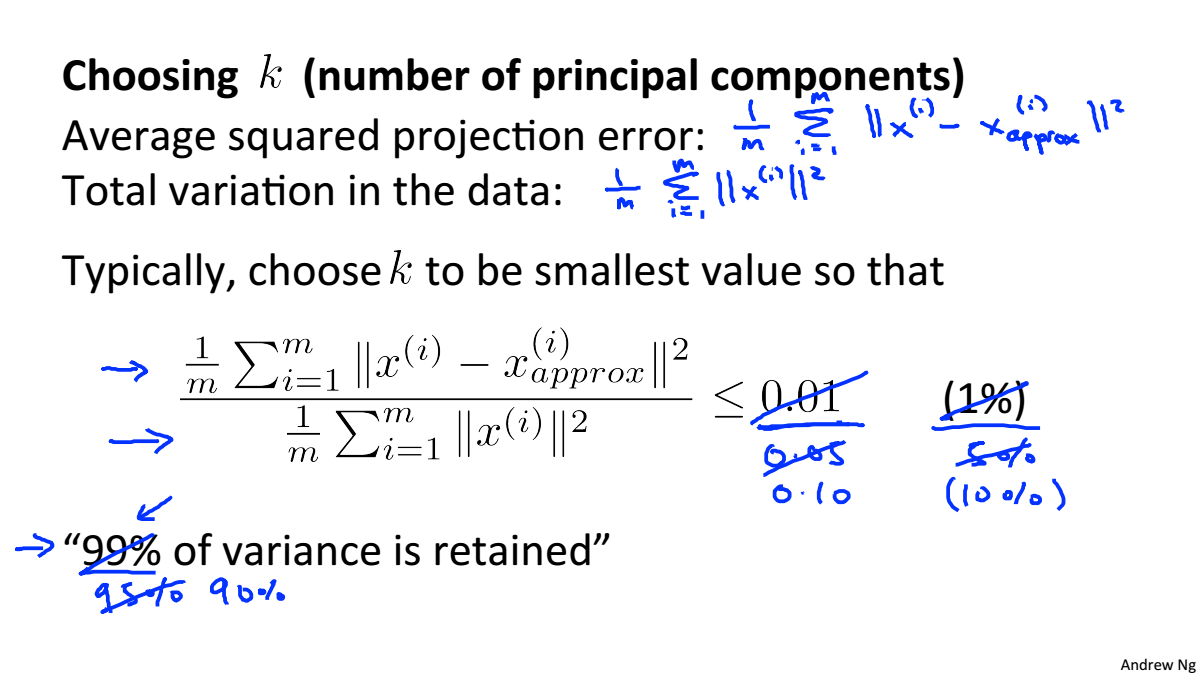选择K的两种两种方法：

1. 尝试不同的K，计算差异性，如果>99%则符合要求
2. 根据svd的到奇异值矩阵，矩阵对角上前K个数的和除以全部数的和就是差异性，从而根据差异性找到K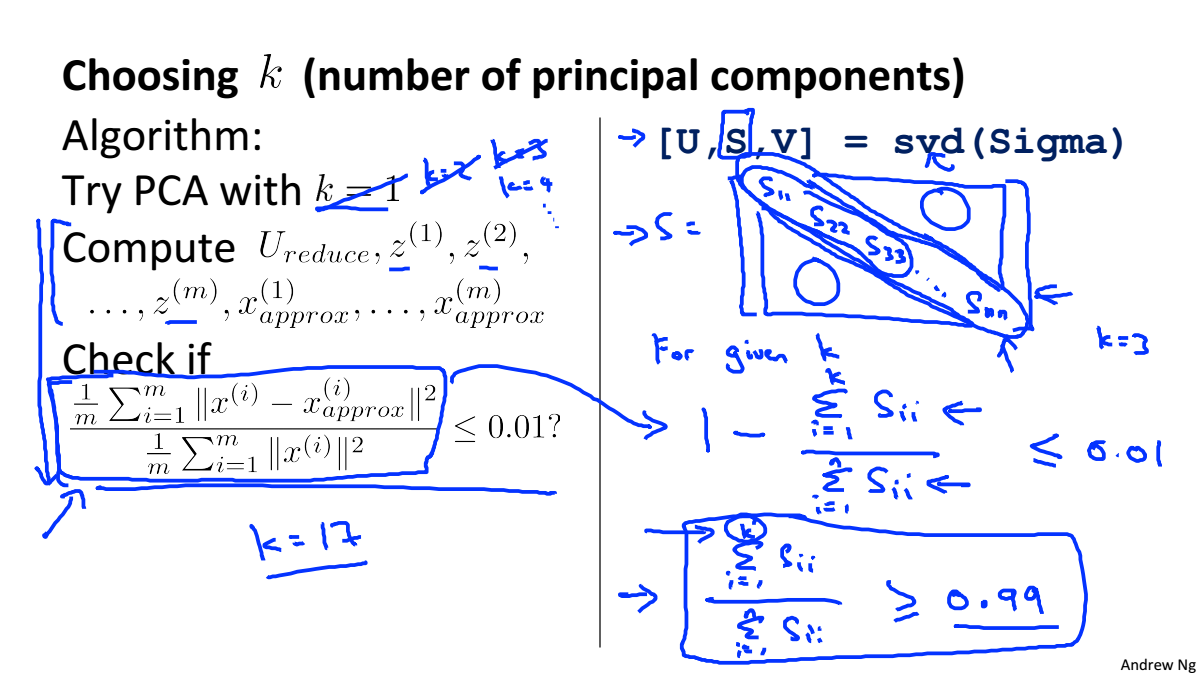监督学习加速
降维矩阵应该在训练集上运行PCA获得，得到降维矩阵后就可以在交叉验证集合测试集上使用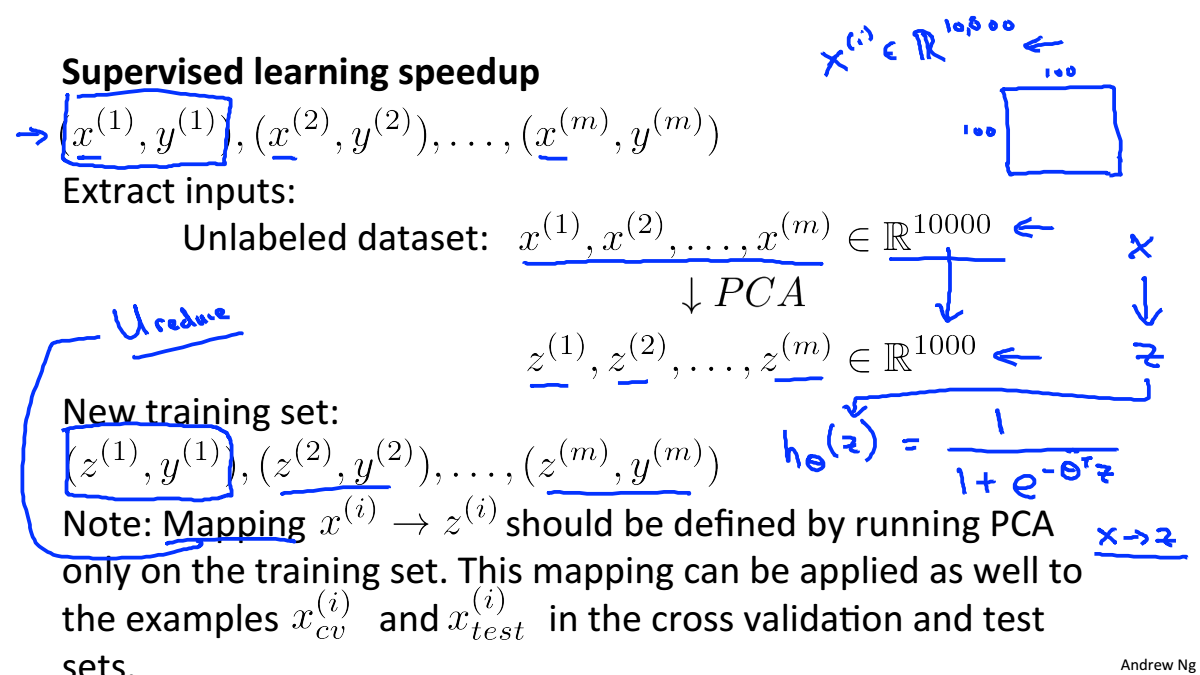PCA并不是一个防止过拟合的好方法，应当使用正则化来防止过拟合k- 机器学习 相关内容

阅读数 6435

博文 来自： qq_34290470

阅读数 12067

博文 来自： databatman
博文 来自： u012965373

阅读数 205

博文 来自： qq_43634001
没有更多推荐了，返回首页